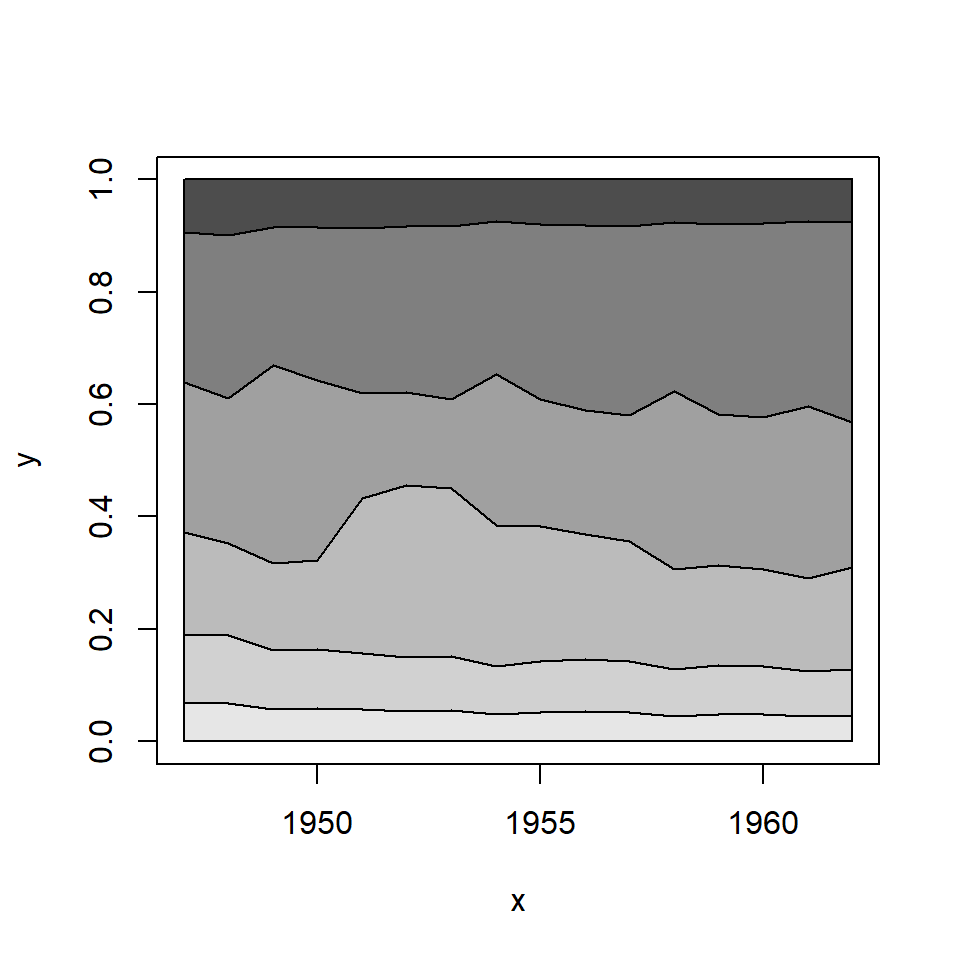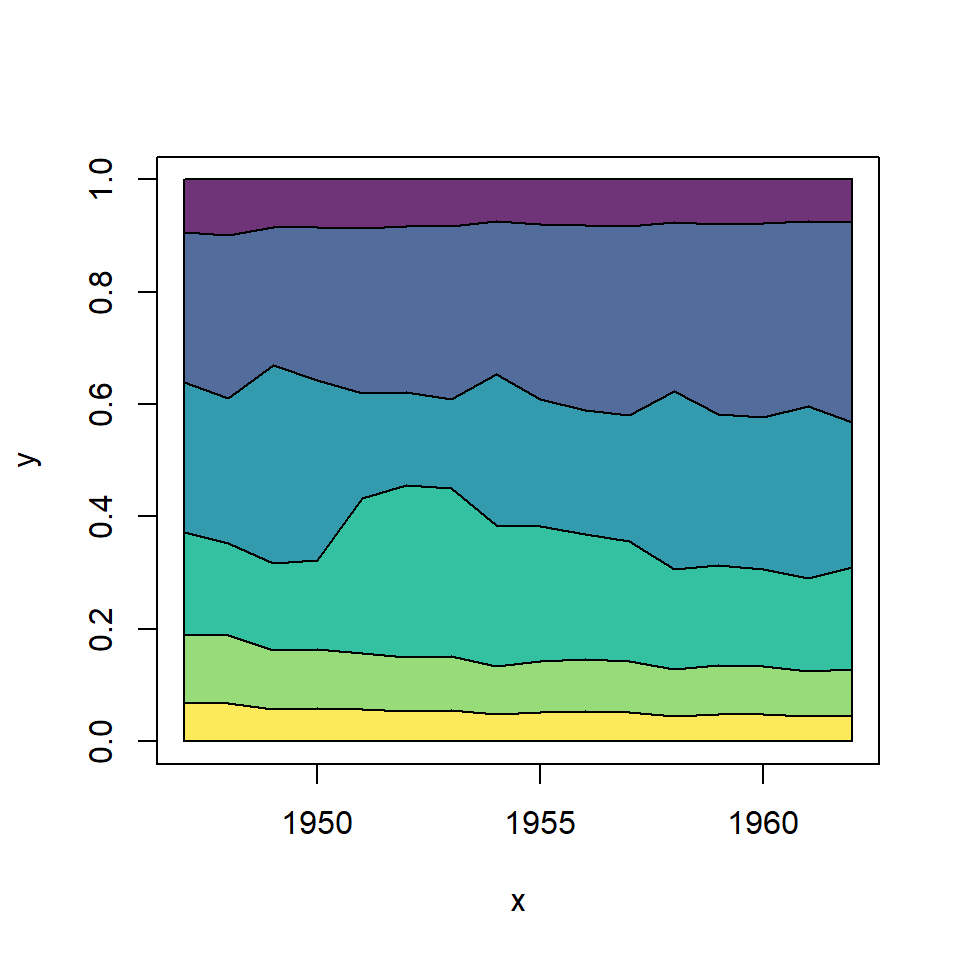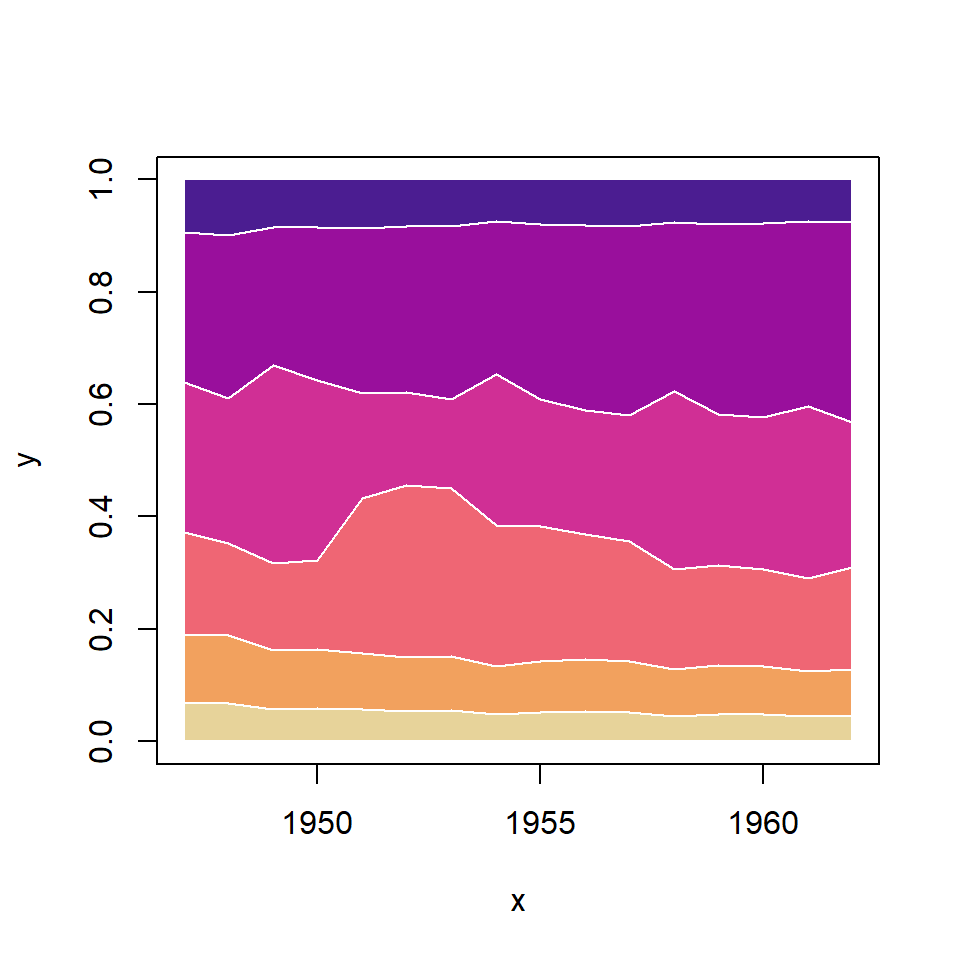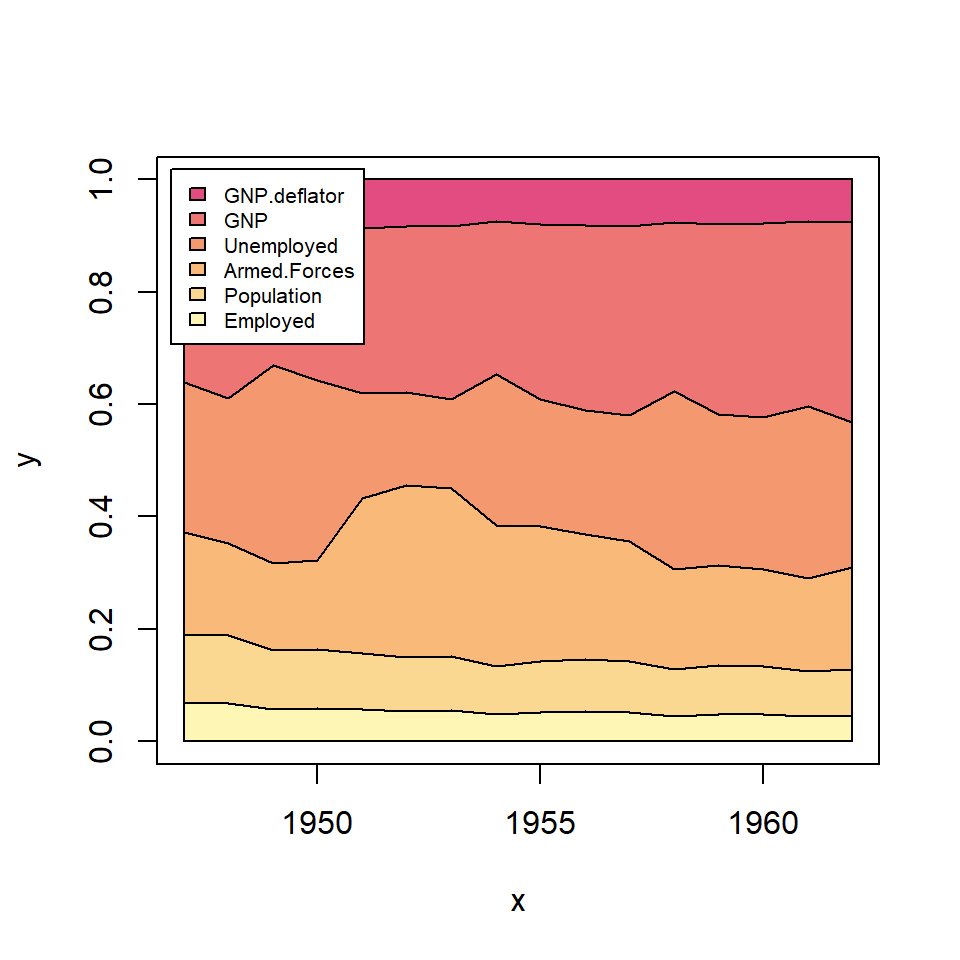# Percentage stacked area chart in R

areaplot

Arni Magnusson

## Sample data set

The data set used in the following examples is a subset of the `longley` data set, where the `x` variable represents the year and the `y` the measure of several variables for each year.

``````# Sample data set
df <- longley

# X-axis variable
x <- df\$Year

# Variables to be stacked
y <- df[, c(1, 2, 3, 4, 5, 7)]``````

## Percentage stacked area chart

In order to create a percentage stacked area plot in R (also known as 100% stacked area chart) you can make use of the `areaplot` function of the package of the same name. You just need to pass your data an set `prop = TRUE`.

``````# install.packages("areaplot")
library(areaplot)

# Percentage stacked area chart
areaplot(x, y, prop = TRUE)

# Equivalent to:
areaplot(. ~ Year, data = df, prop = TRUE)``````## Color customizationYou can pass a vector of colors to the `col` argument to customize the default gray scale. In the following example we are creating a vector of colors of the viridis color palette with a 0.8 level of transparency.

``````# install.packages("areaplot")
library(areaplot)

# Colors
cols <- hcl.colors(6, palette = "viridis", alpha = 0.8)

# Stacked area chart with custom colors
areaplot(x, y, prop = TRUE,
col = cols)``````The function allows passing additional arguments to the `polygon` function (used by the package). This allows customizing the border, line width and line type of the stacked areas.

``````# install.packages("areaplot")
library(areaplot)

# Colors
cols <- hcl.colors(6, palette = "ag_Sunset")

# Stacked area chart with custom borders
areaplot(x, y, prop = TRUE, col = cols,
border = "white",
lwd = 1,
lty = 1)``````

The function also provides a way to add an automatic legend setting `legend = TRUE`. Additional arguments of the `legend` function can be passed through `args.legend` as a list.

``````# install.packages("areaplot")
library(areaplot)

# Colors
cols <- hcl.colors(6, palette = "PinkYl")

areaplot(x, y, prop = TRUE, col = cols,
legend = TRUE,
args.legend = list(x = "topleft", cex = 0.65,
bg = "white", bty = "o"))``````